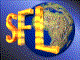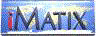| iMatix home page | << | < | > | >>SFLVersion 2.11

### timestamp_string

```#include "sfllang.h"
char *
timestamp_string (char *buffer, const char *pattern)
```

#### Synopsis

Formats a timestamp according to a user-supplied pattern. The result is returned in a buffer supplied by the caller; if this argument is NULL, allocates a buffer and returns that (the caller must then free the buffer using mem_free()). The pattern consists of arbitrary text mixed with insertion symbols indicated by '%':
 %y day of year, 001-366 %yy year 2 digits, 00-99 %yyyy year 4 digits, 0100-9999 %mm month, 01-12 %mmm month, Jan %mmmm month, January %MMM month, JAN %MMMM month, JANUARY %dd day, 01-31 %ddd day of week, Sun %dddd day of week, Sunday %DDD day of week, SUN %DDDD day of week, SUNDAY %w day of week, 1-7 (1=Sunday) %ww week of year, 01-53 %q year quarter, 1-4 %h hour, 00- 23 %m minute, 00- 59 %s second, 00- 59 %c centisecond, 00-99 %% literal character %

#### Source Code - (sfllang.c)

```{
long
date,                           /*  Current date                     */
time;                           /*    and time                       */
int
century,                        /*  Century component of date        */
year,                           /*  Year component of date           */
month,                          /*  Month component of date          */
day,                            /*  Day component of date            */
hour,                           /*  Hour component of time           */
minute,                         /*  Minute component of time         */
second,                         /*  Second component of time         */
centi,                          /*  1/100 sec component of time      */
cursize;                        /*  Size of current component        */
char
*dest,                           /*  Store formatted data here        */
ch;                             /*  Next character in picture        */

date = date now ();
time = time now ();

century = GET_CENTURY (date);
year    = GET_YEAR    (date);
month   = GET_MONTH   (date);
day     = GET_DAY     (date);
hour    = GET_HOUR    (time);
minute  = GET_MINUTE  (time);
second  = GET_SECOND  (time);
centi   = GET_CENTI   (time);

if (buffer == NULL)
buffer = mem_alloc (strlen (pattern) * 2);

/*  Scan through picture, converting each component                      */
dest = buffer;
*dest = 0;                          /*  String is empty                  */
while (*pattern)
{
ch = *pattern++;
if (ch == '%' && *pattern)
{
ch = *pattern++;            /*  Count size of pattern after %    */
for (cursize = 1; *pattern == ch; cursize++)
pattern++;
}
else
{
*dest++ = ch;               /*  Something else - store and next  */
*dest = 0;                  /*  Terminate the string nicely      */
continue;
}

/*  Now process pattern itself                                       */
switch (ch)
{
case 'y':
if (cursize == 1)       /*  y     day of year, 001-366       */
sprintf (dest, "%03d", julian date (date));
else
if (cursize == 2)       /*  yy    year 2 digits, 00-99       */
sprintf (dest, "%02d", year);
else
if (cursize == 4)       /*  yyyy  year 4 digits, 0100-9999   */
sprintf (dest, "%02d%02d", century, year);
break;

case 'm':
if (cursize == 1)       /*  m     minute, 00-59              */
sprintf (dest, "%02d", minute);
else
if (cursize == 2)       /*  mm    month, 01-12               */
sprintf (dest, "%02d", month);
else
if (cursize == 3)       /*  mmm   month, 3 letters           */
strcpy (dest, get month abbrev (month, FALSE));
else
if (cursize == 4)       /*  mmmm  month, full name           */
strcpy (dest, get month name (month));
break;

case 'M':
if (cursize == 3)       /*  MMM   month, 3-letters, ucase    */
strcpy (dest, get month abbrev (month, TRUE));
else
if (cursize == 4)       /*  MMMM  month, full name, ucase    */
{
strcpy (dest, get month name (month));
strupc (dest);
}
break;

case 'd':
if (cursize == 2)       /*  dd    day, 01-31                 */
sprintf (dest, "%02d", day);
else
if (cursize == 3)       /*  ddd   day of week, Sun           */
strcpy (dest, get day abbrev (day of week (date), FALSE));
else
if (cursize == 4)       /*  dddd  day of week, Sunday        */
strcpy (dest, get day name (day of week (date)));
break;

case 'D':
if (cursize == 3)       /*  DDD   day of week, SUN           */
strcpy (dest, get day abbrev (day of week (date), TRUE));
else
if (cursize == 4)       /*  DDDD  day of week, SUNDAY        */
{
strcpy (dest, get day name (day of week (date)));
strupc (dest);
}
break;

case 'w':
if (cursize == 1)       /*  w     day of week, 1-7 (1=Sun)   */
sprintf (dest, "%d", day of week (date) + 1);
else
if (cursize == 2)       /*  ww    week of year, 01-53        */
sprintf (dest, "%d", week of year (date));
break;

case 'q':
if (cursize == 1)       /*  q     year quarter, 1-4          */
sprintf (dest, "%d", year quarter (date));
break;

case 'h':
if (cursize == 1)       /*  h     hour, 00-23                */
sprintf (dest, "%02d", hour);
break;

case 's':
if (cursize == 1)       /*  s     second, 00-59              */
sprintf (dest, "%02d", second);
break;

case 'c':
if (cursize == 1)       /*  c     centisecond, 00-99         */
sprintf (dest, "%02d", centi);
break;

case '%':
if (cursize == 1)       /*  %     literal '%'                */
strcpy (dest, "%");
break;
}
if (*dest)                      /*  If something was output,         */
 | << | < | > | >>Copyright © 1996-2000 iMatix Corporation